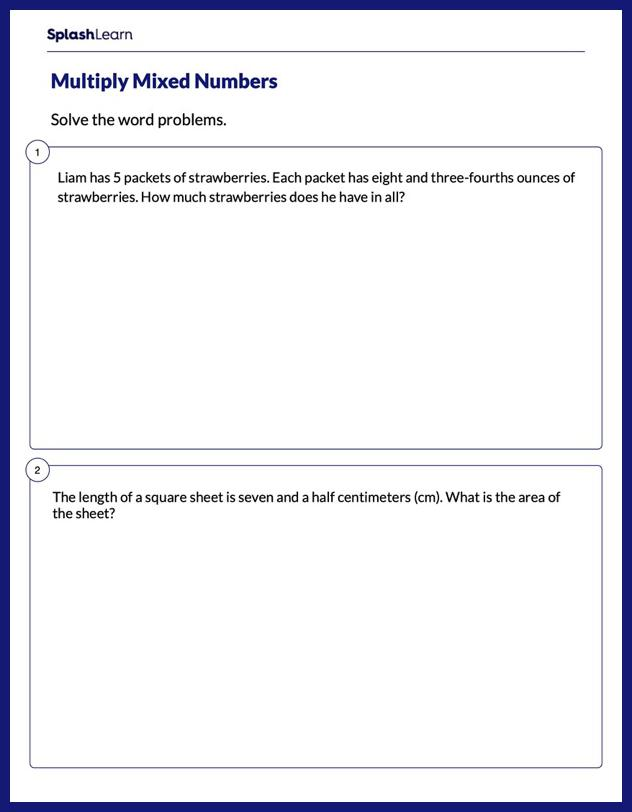# Word Problems on Multiplying Mixed Numbers Worksheet

Home > Word Problems on Multiplying Mixed NumbersBoost your child's understanding of fractions with this worksheet. Students will work with a set of word problems on multiplication of mixed numbers . They will make sense of multiply by scenarios and find the unknown quantity. This worksheet will nudge your young mathematician toward mastery and develop their all round mathematical acumen.